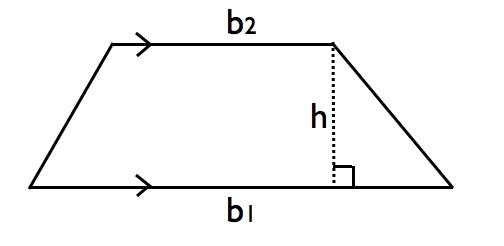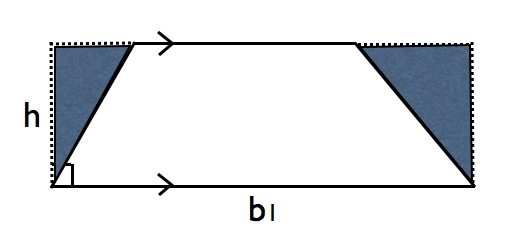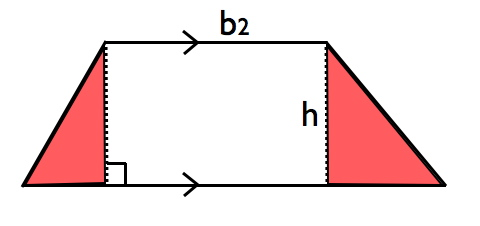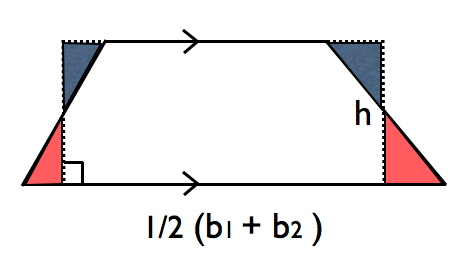Learn the 4 Things You Must Know Before Taking the GMAT!   Download it Now!

For whatever reason, trapezoids tend to freak students out on the GMAT. Maybe it’s because the formula for finding the area of a trapezoid doesn’t “look” like all the other quadrilateral formulas. Maybe it’s because trapezoids have a bizarre looking shape. Whatever the reason, I’d like to make your life a whole lot easier as you continue with your GMAT prep and work through your Dominate the GMAT course.

Specifically, I’d like to help you think through what the area of a trapezoid is in a way that will make sense to you. See, most GMAT problem solving and data sufficiency questions that involve trapezoids generally boil down to your ability to properly apply the formula for the area of a trapezoid. Yet, as I’ve said so often before, you do yourself a disservice if you try to cram a bunch of formulas into your head. You’ll simply become mentally overwhelmed on test day, and you’ll start to freak out if you can’t quite recall a specific formula (or worse, if you confuse it with another one buried deep in the recesses of your brain). Fortunately for you, there’s a better way.

First things first. What is a trapezoid? A trapezoid is a four-sided geometric figure (i.e. a quadrilateral) where two of the sides are parallel, and two are not (see figure below). The two parallel sides are called bases. The height of a trapezoid is simply the altitude, as with triangles (see instructional video GMAT Geometry – Triangles). You may need to draw the altitude if one of the sides isn’t already perpendicular to the bases.The question then becomes, what is the area of this trapezoid? Let me make your life very simple. A helpful thing to remember on test day is that the area of a quadrilateral is always base times height. A = b*h. Very simple.

Now you may be saying, “But Brett, wait! That’s not the formula for the area of a trapezoid that I remember seeing!” Well, it is and it isn’t. Let me explain.

The formula for the area of a trapezoid is still A = b*h. The question is, which base do you use? See, the problem with trapezoids is that they have two bases, each of which is a different length. With rectangles, for example, it’s easy — either base you pick, by definition, is the same length as the parallel side opposite it (i.e. the other base). Same with squares. Same with parallelograms. But not so for trapezoids.

Look at this figure. If we choose the longer base, in this case b1, what happens when we multiply b1 * h? The resulting area is too large! (notice the blue triangles are surplus area, beyond the borders of the trapezoid itself).Okay, so then we must choose the shorter base, right? Well, as you can see, we have the opposite problem. The resulting area is too small by the amount of the red triangles.There’s a very simple solution. If the longer base produces an area that’s too large, and the smaller base produces an area that’s too small, what if we just take the average of the two bases? Sure enough, that’s exactly what you’re supposed to do. Graphically, you can see how averaging the bases produces an area that perfectly balances the surplus created by the larger bases (blue shaded regions) and the shortfall created by the shorter base (red shaded regions).So, while the formula for the area of a trapezoid may have looked weird to you when you first read it in a textbook, hopefully now you see that it’s really just base times height — except the “base” we use is the average of the two bases [1/2(b1+b2)].

To summarize:

Area of a Trapezoid  = 1/2 (b1 + b2) * h

Now that you know that, you should be able to solve most trapezoid questions on GMAT problem solving and GMAT data sufficiency questions. In your GMAT Review Official Guide, 12th Ed., there’s a great application example on p.172, #145. Give it a try and see how you do. (Hint: You’ll need to apply some of what you know about right triangles as well). The answer explanation is on p.232. As always, don’t hesitate to e-mail me ([email protected]) with questions.

Internalize this approach so that you can go out and dominate the GMAT!